PROC RSREG Statement
PROC RSREG <options> ;

The PROC RSREG statement invokes the procedure. You can specify the following options in the PROC RSREG statement.

DATA=SAS-data-set

specifies the input SAS data set that contains the data to be analyzed. By default, PROC RSREG uses the most recently created SAS data set.

NOPRINT

suppresses the normal display of results when only the output data set is required.

For more information, see the description of the NOPRINT option in the MODEL and RIDGE statements.

Note that this option temporarily disables the Output Delivery System (ODS); see Chapter 20, Using the Output Delivery System, for more information.

OUT=SAS-data-set

creates an output SAS data set that contains statistics for each observation in the input data set. In particular, this data set contains the BY variables, the ID variables, the WEIGHT variable, the variables in the MODEL statement, and the output options requested in the MODEL statement. You must specify output statistic options in the MODEL statement; otherwise, the output data set is created but contains no observations. To create a permanent SAS data set, you must specify a two-level name (see SAS Language Reference: Concepts for more information about permanent SAS data sets). For more details, see the section OUT=SAS-data-set.

PLOTS <(global-plot-option)>=plot-request<(options)>
PLOTS <(global-plot-option)>=(plot-request<(options)><plot-request<(options)>>)

controls the plots produced through ODS Graphics. When you specify only one plot-request, you can omit the parentheses from around the plot-request. For example:

```plots = all
plots = (diagnostics ridge surface(unpack))
plots(unpack) = surface(overlaypairs)
```

ODS Graphics must be enabled before requesting plots. For example:

```ods graphics on;
proc rsreg plots=all;
model y=x;
run;
ods graphics off;
```

For more information about enabling and disabling ODS Graphics, see the section Enabling and Disabling ODS Graphics in Chapter 21, Statistical Graphics Using ODS.

By default, no graphs are created; you must specify the PLOTS= option to make graphs. See Figure 78.4, Output 78.1.5, Output 78.1.6, Output 78.2.3, and Output 78.2.4 for examples of the ODS graphical displays.

The following global-plot-option is available.
UNPACKPANELS | UNPACK

suppresses paneling. By default, multiple plots can appear in some output panels. Specify the UNPACK option to display each plot separately.

The following plot-requests are available.
ALL

produces all appropriate plots. You can specify other options with ALL; for example, to display all plots and unpack the SURFACE contours you can specify plots=(all surface(unpack)).

DIAGNOSTICS <(LABEL | UNPACK )>

displays a panel of summary fit diagnostic plots. The plots produced and their usage are discussed in Table 78.1.

Table 78.1 Diagnostic Plots

Diagnostic Plot

Usage

Cook’s D statistic versus observation number

Evaluate influence of an observation on the entire parameter estimate vector

Dependent variable values versus predicted values

Evaluate adequacy of fit and detect influential observations

Externally studentized residuals (RStudent) versus leverage

Detect outliers and influential (high-leverage) observations

Externally studentized residuals versus predicted values

Evaluate adequacy of fit and detect outliers

Histogram of residuals

Confirm normality of error terms

Normal quantile plot of residuals

Confirm normality and homogeneity of error terms, and detect outliers

Residuals versus predicted values

Evaluate adequacy of fit and detect outliers

side-by-side quantile plots of the centered fit and the residuals show "how much variation in the data is explained by the fit and how much remains in the residuals" (Cleveland; 1993)

Observations satisfying RStudent > 2 or RStudent < –2 are called outliers, and observations with leverage > 2p/n are called influential, where n is the number of observations used in fitting the model and p is the number of parameters used in the model (Rawlings, Pantula, and Dickey; 1998). Specifying the LABEL option labels the influential and outlying observations—the label is the first ID variable if the ID statement is specified; otherwise, it is the observation number. Note in the Cook’s D plot that only observations with D exceeding 4/n are labeled; these are also called influential observations. The UNPACK option displays each diagnostic plot separately. See Output 78.2.3 for an example of the diagnostics panel.

FIT <(GRIDSIZE=number)>

plots the predicted values against a single predictor when you have only one factor or only one covariate in the model. The GRIDSIZE= option specifies the number of points at which the fitted values are computed; by default, GRIDSIZE=200.

NONE

suppresses all plots.

RESIDUALS <(UNPACK | SMOOTH)>

displays plots of residuals against each factor and covariate. The UNPACK option displays each residual plot separately. The SMOOTH option overlays a loess smooth on each residual plot; see Chapter 52, The LOESS Procedure, for more information. See Output 78.1.5 for an example of this plot.

RIDGE <(UNPACK)>

displays the maximum and/or minimum ridge plots. This option is available only when a MAXIMUM or MINIMUM option is specified in the RIDGE statement. The UNPACK option displays the estimated response and factor level ridge plots separately. See Output 78.1.5 for an example of this plot.

SURFACE <(surface-options)>

displays the response surface for each response variable and each pair of factors with all other factors and covariates fixed at their means. By default a panel of contour plots is produced; see Output 78.1.6 for an example of this plot. The following surface-options can be specified:

3D

displays three-dimensional surface plots instead of contour plots. See Figure 78.4 for an example of this plot.

AT <keyword><(variable=value-list | keyword <...variable=value-list | keyword>)>

specifies fixed values for factors and covariates. You can specify one or more numbers in the value-list or one of the following keywords:

 MIN sets the variable to its minimum value. MEAN sets the variable to its mean value. MIDRANGE sets the variable to the middle value: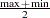. MAX sets the variable to its maximum value.

Specifying a keyword immediately after AT sets the default value of all variables; for example, AT MIN sets all variables not displayed on an axis to their minimum values. By default, continuous variables are set to their means (AT MEAN) when they are not used on an axis. For example, if your model contains variables X1, X2, and X3, then specifying AT(X1=7 9) produces a contour plot of X2 versus X3 fixing X1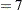and then another contour plot with X1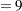, along with contour plots of X1 versus X2 fixing X3 at its mean, and X1 versus X3 fixing X2 at its mean.

EXTEND=value

extends the surface value-times the range of each factor in each direction, which enables you to see more of the fitted surface. For example, if factor A has range
, then specifying EXTEND=0.1 will compute and display the surface for A in
. You can specify value
; by default, value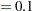.

FILL=PRED | SE | NONE

produces a filled contour plot for either the predicted values or the standard errors. FILL=SE is the default. If the 3D option is also specified, then the contour plot is projected onto the surface.

GRIDSIZE=n

creates an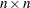grid of points at which the estimated values for the surface and standard errors are computed, for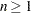. By default,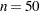.

LINE<=PRED | SE | NONE>

produces a contour line plot for either the predicted values or the standard errors. LINE=PRED is the default. If the 3D option is also specified, then specifying LINE displays a grid on the surface, and the other LINE= specifications are ignored.

NODESIGN

suppresses the display of the design points on the contour surface plots and the overlaid contour-line plots.

OVERLAYPAIRS

produces overlaid contour line plots for all pairs of response variables in addition to the contour surface plots. See Figure 78.6 for an example of this plot.

ROTATE=angle

rotates the 3-D surface plots angle degrees, –180 < angle < 180. By default, angle = 57.

TILT=angle

tilts the 3-D surface plots angle degrees, –180 < angle < 180. By default, angle = 20.

UNPACKPANELS | UNPACK

suppresses paneling, and displays each surface plot separately.